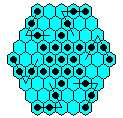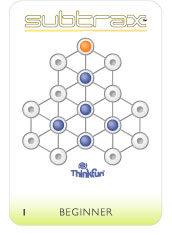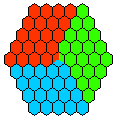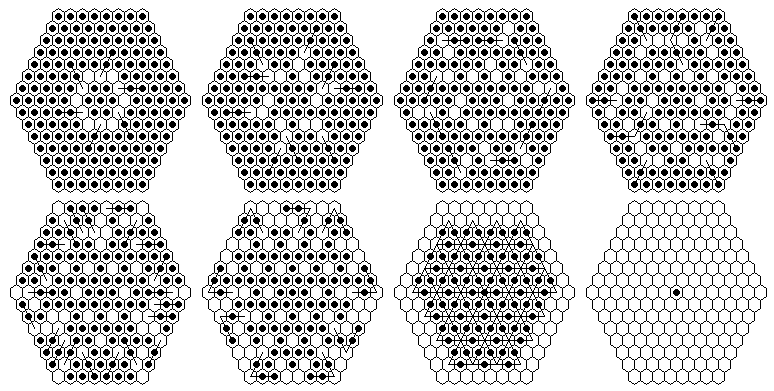# Peg Solitaire on Hexagonal Boards

## Hexagonal BoardsHexagonal boards are the analog of square boards in the triangular lattice world. A hexagonal board of side n, will be referred to as Hexagon(n). This board has 6T(n-1)+1 = 3n(n-1)+1 holes. The board Hexagon(n) is a null-class board if (and only if) n is of the form 3i+2, so n=2, 5, 8, etc (this is equivalent to requiring that the diameter be a multiple of 3). Hexagonal boards always have a center hole. If a hexagonal board is not a null-class board, then the full board is in the same position class as a peg at the central hole. Therefore on such boards no single vacancy to single survivor problem is solvable which begins or ends at the center. The same notation as for triangular boards is used, where the leftmost hole on the top row is "a1".Hexagon(1) and Hexagon(2) boards are uninteresting. The Hexagon(3) board has 19 holes and is not null-class. Although several single vacancy to single survivor problems would appear possible by considering position classes, in fact none are solvable. This can be proven by appropriate resource count and exit arguments, first demonstrated by Michael Merchant in 1976 [B2].

Subtrax is a peg solitaire game marketed by ThinkFun, it was designed by puzzle expert Nob Yoshigahara. The board is Hexagon(3) with three symmetric corner holes removed. This board is not null-class, and it is not clear to me why the three corners were removed. Most of the problems are small patterns, so the shape of the board is not that important.The 37-hole Hexagon(4) board is not null-class, but is the smallest hexagonal board on which a single vacancy to single survivor problem is solvable. The diagram to the left shows a finishing 16-sweep (starting at f7 and ending at b1), together with the complement of this position on the right. This sweep can be reached from a single vacancy start. You can show this by playing from the complement position to a single peg, a fairly difficult problem to work out on a Chinese Checkers board. This is the longest finishing sweep possible on this board.A game marketed by Pressman Toy Corporation under the name "Think And Jump" is Hexagon(4) with six of the edge jumps not allowed, forming a "snowflake" pattern. This board is still not null-class, and the removal of jumps makes it more difficult. Since the directions describe starting from the central vacancy, the problem as stated has no solution. However if we apply the usual diagonal labeling where the center is labeled "1", it is possible to solve problems of the form: "Vacate 2, finish at 3" or vice versa.

The 61-hole Hexagon(5) board is the smallest hexagonal board where a complement problem is solvable, and in fact all complement problems on this board are solvable. This is also the smallest (gapless) board with 12-fold symmetry where the central complement problem is solvable. A solution to the central complement problem on this board can pass through board states with 6-fold rotational symmetry, a snapshot from such a solution is shown in the blue diagram above, with the full solution shown below.Hexagonal boards have a high degree of symmetry. One interesting property of the null-class hexagonal board is that it can be cut like a pie into three identical pieces, where each piece contains the center hole and is also null-class. Each piece is an n by n-1 rectangle with one extra hole for a total of n(n-1)+1 holes.

The left diagram shows this decomposition for the Hexagon(5) board, the central hole in this case is e5. We can then take any solution to the e5-complement problem on the small board, and duplicate it twice (at appropriate times after e5 is cleared) to clear the other sections. For example, consider the following solution on the red board:By modifying the three finishing moves, we can construct a solution to the e5-complement on the hexagonal board. The diagram below shows the full 32-move solution on Hexagon(5):What is the shortest solution to the "central game" or e5-complement? The shortest known solution has 21 moves (example below), but it is possible that it could be solved in 20 or even 19 moves.It is also interesting to find solutions to the central game which go through positions of 6-fold and 3-fold symmetry, or finish with long sweeps.

A solution on Hexagon(5) that finishes with a 9-sweep:A solution on Hexagon(8) that finishes with an 84-sweep. The first 11 moves are the same as in the previous diagram, and take you to a board position with 6-fold symmetry. Only the moves after this are shown in the diagram below, which remarkably can clear the entire board in only 8 more diagrams:Although it is not on a hexagonal board, the solution below on Star(5) is quite similar to the last solution shown for Hexagon(5). As before the first 11 moves are not shown as they are exactly the same.## Board Symmetry

In square lattice peg solitaire, it is interesting to take all boards with square symmetry, and determine which are null-class [P3]. A similar thing can be done in triangular peg solitaire. On a hexagonal lattice, the highest degree of symmetry is 12-fold. One can represent all gapless boards with 12-fold symmetry as

Hexagon(n) + (mi)

where the sequence mi represents the six rows of holes of length mi added symmetrically around the hexagon. The board Star(n) in this notation is Hexagon(n) + (n-1, n-2, ... , 1). The general requirements on the finite sequence mi are that it must be decreasing, the terms must alternate in parity, and m1 must be less than n and of opposite parity. Such notation can be used to (uniquely) describe any gapless board with 12-fold symmetry. An important point is that the sequence mi must be decreasing (rather than non-increasing), which means we cannot have arbitraily long needle-like projections as in the square lattice case.

Theorem: The board Hexagon(n) + (mi) is null-class if and only if n is of the form 3i+2 (in other words n must have a remainder of 2 when divided by 3, or n=2 (mod 3)).

This is easily proved by a similar argument as in the square lattice case, by showing that board locations are always added in pairs with the same parity labels.

We can then consider the problem of solving all complement problems on these null-class boards. As in [P3], a board where the complement problem is solvable at every board location is called universally solvable.

Conjecture: All gapless boards in a triangular lattice with 12-fold symmetry are universally solvable except for Hexagon(2) and Hexagon(2) + (1) = Star(2).

This is quite a different situation than the square lattice case, where most square-symmetric boards are not universally solvable.Triangular Peg Solitaire Main Page ...